# FEM-based shakedown analysis of hardening structures

Open Access
Research

## Abstract

This paper develops a new finite element method (FEM)-based upper bound algorithm for limit and shakedown analysis of hardening structures by a direct plasticity method. The hardening model is a simple two-surface model of plasticity with a fixed bounding surface. The initial yield surface can translate inside the bounding surface, and it is bounded by one of the two equivalent conditions: (1) it always stays inside the bounding surface or (2) its centre cannot move outside the back-stress surface. The algorithm gives an effective tool to analyze the problems with a very high number of degree of freedom. Our numerical results are very close to the analytical solutions and numerical solutions in literature.

## Keywords

Ratchetting Kinematic hardening Two-surface plasticity Shakedown FEM

## Background

Shakedown analysis for hardening structures has been investigated by many researchers. Among hardening models, the isotropic hardening law is generally not reasonable in situations where structures are subjected to cyclic loading because it does not account for the Bauschinger effect and rejects the possibility of incremental plasticity. The unbounded kinematic hardening model has already been introduced theoretically by Melan  and later by Prager . Applications of this model have been investigated by Maier  and Ponter . The unbounded kinematic hardening model cannot estimate the plastic collapse and also incremental plasticity but only low-cycle fatigue, while low-cycle fatigue limit with the kinematical hardening model seems not to be essentially different from the perfectly plastic model, cf. Gokhfeld and Cherniavsky  and Stein and Huang .

Introducing a bounding surface in Melan-Prager's model, a two-surface model of plasticity with a fixed bounding surface is achieved which appears to be most basic, suitable and simple for shakedown analysis. Application of bounded kinematic hardening model was introduced theoretically and numerically by Weichert and Groß-Weege  who used the generalized standard material model (GSM). They used Airy's stress function to satisfy the equilibrium conditions in the interior of the structures fulfilled. Shakedown theorems for bounded linear and nonlinear kinematic hardening have been proposed by Bodovillé and de Saxcé , Pham [9, 10] and Nguyen .

Numerical investigations for bounded kinematic hardening using basic reduction technique have been introduced by Staat and Heitzer [12, 13] and Stein and Zhang . By the lower bound approach, it permits to avoid the nondifferentiability of the objective function, which must be regularized via internal dissipation energy and there is no incompressibility constraint in nonlinear programming problem, but this approach suffers from nonlinear inequality constraints.

A company of lower bound algorithm is the upper bound algorithm, which is based on Koiter theorem. For perfectly plastic structures, the upper bound algorithm has been established by Yan and Nguyen Dang [15, 16] and Yan et al. . The major numerical obstacle in this approach is the singular property of plastic dissipation function. Dealing with this difficulty, the researchers replaced the original dissipation function ${\mathit{D}}^{\mathit{p}}\left({\stackrel{˙}{\mathit{ϵ}}}_{\mathit{ij}}^{\mathit{p}}\right)$ by ${\mathit{D}}^{\mathit{p}}\left({\stackrel{˙}{\mathit{ϵ}}}_{\mathit{ij}}^{\mathit{p}}+{\mathit{ϵ}}_{0}^{2}\right)$, where ϵ0 is a very small number. This technique is also used in our algorithm.

By using the static approach and the criterion of the mean, Nguyen Dang and König  showed that the shakedown solution can be obtained by a maximization or a minimization problem. The yield criterion of the mean was further applied in practical computations by displacement method and equilibrium finite element by Nguyen Dang and Palgen .

A very efficient primal-dual algorithm, which can derive lower and upper bound simultaneously of shakedown limit load factor for complicated structures, has been introduced by Vu, Yan and Nguyen Dang [20, 21, 22] and Vu . In these works, dual relationship between upper bound and lower bound for shakedown analysis of perfectly plastic structures has been proven. Theoretically speaking, primal-dual algorithm helps to find a very accurate solution of shakedown analysis problem.

While using the finite element method (FEM) for limit and shakedown analysis, the stress method can be used, but this method is restricted since for certain structures, it is very difficult to find appropriate stress function, so the displacement method is preferred to make the numerical approach as general as possible.

For the structures with hardening material, it is difficult to prove the relationship between upper bound and lower bound because of the complication of the objective function. Furthermore, in the static approach, it is difficult to present alternating limit and ratcheting limit separately. In this paper, we have presented a FEM-based upper bound algorithm for shakedown analysis of bounded kinematic hardening structures with von Mises yield criterion. By the direct plasticity methods, shakedown analysis is a nonlinear programming problem. The present algorithm can deal with complicated realistic structures which are modelled by 3D, 20-node elements with huge number of degree of freedom. Two numerical examples are included to validate the algorithm and to study the influence of hardening effect.

## Methods

### Bounded kinematic hardening model

For kinematic hardening model, the initial yield surface can translate in the multi-axial stress space, without changing its shape and size. If the translation is unlimited, or in other words, the ultimate strength of material σ u is infinite, we have unbounded model (Figure 1). This model is inadequate to predict the plastic collapse (both incremental and instantaneous) of structure. It can only describe the alternating plasticity mode.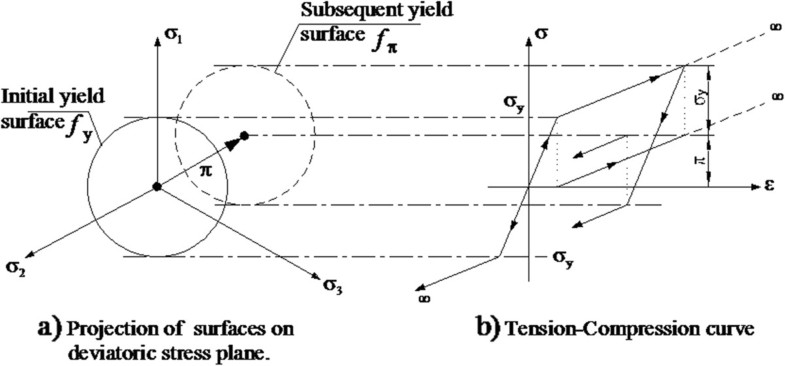Figure 1 Unbounded kinematic hardening model.
The initial yield surface for von Mises material is defined as below
$\mathit{F}\left[\mathbf{\sigma }\right]-{\mathit{\sigma }}_{\mathit{y}}^{2}=0.$
(1)
The subsequent surface is defined as
$\mathit{F}\left[\mathbf{\sigma }-\mathbf{\pi }\right]-{\mathit{\sigma }}_{\mathit{y}}^{2}=0$
(2)

where π is the back stress. If hardening is unbounded, π is infinite.

For more realistic material, yield stress σ y must be bounded by ultimate strength σ u . A simple two-surface model is used to model the bounded hardening. The subsequent yield surface may or may not touch the fixed bounding surface; see Figure 2. This is satisfied by one of the two following conditions:
$\mathit{F}\left[\mathbf{\sigma }\right]\le {\mathit{\sigma }}_{\mathit{u}}^{2}.$
(4)
1. 1.
Centre of subsequent yield surface cannot move outside the back-stress surface. This is expressed by
$\mathit{F}\left[\mathbf{\pi }\right]\le {\left({\mathit{\sigma }}_{\mathit{u}}-{\mathit{\sigma }}_{\mathit{y}}\right)}^{2}.$
(3)

2. 2.

Subsequent yield surface always stays inside bounding surface. This is expressed by

In the preceding conditions, Equations 3 and 4, equalities occur when the subsequent surface touches bounding surface. We have proven that bounding conditions (3) and (4) are exactly equivalent. See detail in the study of Pham and Staat .

### Shakedown formulation based on Koiter's theorem

#### Problem establishment

Upper bound solution of shakedown load multiplier is the solution of a constrained nonlinear programming problem
$\begin{array}{ll}{\mathit{\alpha }}_{\mathrm{sd}}=\underset{{\stackrel{˙}{\mathbf{ϵ}}}^{\mathit{p}}}{min}{\int }_{0}^{\mathit{T}}{\int }_{\mathit{V}}{\mathit{D}}^{\mathit{p}}\left({\stackrel{˙}{\mathbf{ϵ}}}^{\mathit{p}}\right)\mathit{dV}\mathit{dt}& \left(\mathrm{a}\right)\\ \mathrm{s}.\mathrm{t}.:\left\{\begin{array}{ll}\mathit{\Delta }{\mathbf{ϵ}}^{\mathit{p}}={\int }_{0}^{\mathit{T}}{\stackrel{˙}{\mathbf{ϵ}}}^{\mathit{p}}\mathit{dt}& \phantom{\rule{3em}{0ex}}\left(\mathrm{b}\right)\\ \mathrm{tr}\left({\stackrel{˙}{\mathbf{ϵ}}}^{\mathit{p}}\right)=0& \phantom{\rule{3em}{0ex}}\left(\mathrm{c}\right)\\ \mathit{\Delta }{\mathbf{ϵ}}^{\mathit{p}}=\frac{1}{2}\left(\nabla \left(\mathit{\Delta }\mathbf{u}\right)+\nabla {\left(\mathit{\Delta }\mathbf{u}\right)}^{\mathit{T}}\right)& \phantom{\rule{0.75em}{0ex}}\mathrm{in}\phantom{\rule{0.25em}{0ex}}\mathit{V}& \phantom{\rule{3em}{0ex}}\left(\mathrm{d}\right)\\ \mathit{\Delta }\mathbf{u}=\mathbf{0}& \mathrm{on}\phantom{\rule{0.25em}{0ex}}\partial {\mathit{V}}_{\mathit{u}}& \phantom{\rule{3em}{0ex}}\left(\mathrm{e}\right)\\ {\int }_{0}^{\mathit{T}}{\int }_{\mathit{V}}{\mathbf{\sigma }}^{\mathit{E}}\left(\mathbf{x},\mathit{t}\right):{\stackrel{˙}{\mathbf{ϵ}}}^{\mathit{p}}\mathit{dVdt}=1& \phantom{\rule{3em}{0ex}}\left(\mathrm{g}\right)\end{array}\right\\end{array}$
(5)
where total plastic energy dissipation ${\mathit{D}}^{\mathit{p}}\left({\stackrel{˙}{\mathbf{ϵ}}}^{\mathit{p}}\right)$ in the structure is as follows:
${\int }_{0}^{\mathit{T}}{\int }_{\mathit{V}}{\mathit{D}}^{\mathit{p}}\left({\stackrel{˙}{\mathbf{ϵ}}}^{\mathit{p}}\right)\mathit{dVdt}={\int }_{0}^{\mathit{T}}{\int }_{\mathit{V}}\sqrt{\frac{2}{3}}\phantom{\rule{0.25em}{0ex}}{\mathit{\sigma }}_{\mathit{y}}∥{\stackrel{˙}{\mathbf{ϵ}}}^{\mathit{p}}∥\mathit{dVdt}+{\int }_{\mathit{V}}\sqrt{\frac{2}{3}}\left({\mathit{\sigma }}_{\mathit{u}}-{\mathit{\sigma }}_{\mathit{y}}\right)∥\mathit{\Delta }{\mathbf{ϵ}}^{\mathit{p}}∥\mathit{dV}$
(6)

The first term in the right hand side of Equation 6 is plastic energy dissipation of perfect plasticity material, and the second term is hardening effect. Evidently, if σ u  = σ y , we have ideal plastic material.

Constraint (5b) is the definition of plastic strain accumulation. The plastic strain rate ${\stackrel{˙}{\mathbf{ϵ}}}^{\mathit{p}}$ may not necessarily be compatible, but Δ ϵ p must be compatible. This is expressed by constraints (5d) and (5e). Constraint (5c) is the incompressibility condition, and (5g) is the normalized condition.

#### Problem discretization

Based on FEM, whole structure V is discretized into ne finite elements with NG = ne × ng Gaussian points, where ng is number of Gaussian points in each element. If the load domain is convex, it is sufficient to check if shakedown will happen at all vertices of . So the load domain can be discretized into finite number of load combinations ${\stackrel{^}{\mathbf{P}}}_{\mathit{k}}$, k = 1, …, m, and m is total number of vertices of . By these discretizations, the shakedown analysis is reduced to checking shakedown conditions at all Gaussian points and all load vertices m, instead of checking for whole structure V and entire load domain . Then, numerical form of Equation 5 is as follows:
$\begin{array}{l}{\mathit{\alpha }}_{\mathrm{sd}}^{\mathrm{blkh}}=\underset{{\stackrel{˙}{\mathbf{ϵ}}}^{\mathit{p}}}{min}\left\{\sqrt{\frac{2}{3}}{\mathit{\sigma }}_{\mathit{y}}\sum _{\mathit{k}=1}^{\mathit{m}}\sum _{\mathit{i}=1}^{\mathrm{NG}}\sqrt{{\mathit{w}}_{\mathit{i}}^{2}{\mathbf{ϵ}}_{\mathit{ik}}^{\mathit{T}}\mathbf{D}{\mathbf{ϵ}}_{\mathit{ik}}+{\mathit{w}}_{\mathit{i}}^{2}{\mathit{ϵ}}_{0}^{2}}\right\+\sqrt{\frac{2}{3}}\left({\mathit{\sigma }}_{\mathit{u}}-{\mathit{\sigma }}_{\mathit{y}}\right)\sum _{\mathit{i}=1}^{\mathrm{NG}}\sqrt{{\mathit{w}}_{\mathit{i}}^{2}\sum _{\mathit{k}=1}^{\mathit{m}}{\mathbf{ϵ}}_{\mathit{ik}}^{\mathit{T}}\mathbf{D}\sum _{\mathit{k}=1}^{\mathit{m}}{\mathbf{ϵ}}_{\mathit{ik}}+{\mathit{w}}_{\mathit{i}}^{2}{\mathit{ϵ}}_{0}^{2}}}\phantom{\rule{1.25em}{0ex}}\left(\mathrm{a}\right)\\ \mathrm{s}.\mathrm{t}.:\\ \left\{\begin{array}{lll}\sum _{\mathit{k}=1}^{\mathit{m}}{\mathbf{ϵ}}_{\mathit{ik}}={\mathbf{B}}_{\mathit{i}}\mathbf{u}& \forall \mathit{i}=\overline{1,\mathrm{NG}}& \phantom{\rule{6em}{0ex}}\left(\mathrm{b}\right)\\ {\mathbf{D}}_{\mathit{M}}{\mathbf{ϵ}}_{\mathit{ik}}=\mathbf{0}& \forall \mathit{i}=\overline{1,\mathrm{NG}}\phantom{\rule{1.25em}{0ex}}\forall \mathit{k}=\overline{1,\mathit{m}}& \phantom{\rule{6em}{0ex}}\left(\mathrm{c}\right)\\ \sum _{\mathit{k}=1}^{\mathit{m}}\sum _{\mathit{i}=1}^{\mathrm{NG}}{\mathit{w}}_{\mathit{i}}{\mathbf{ϵ}}_{\mathit{ik}}^{\mathit{T}}{\mathbf{\sigma }}_{\mathit{ik}}^{\mathit{E}}=1& \phantom{\rule{4em}{0ex}}\left(\mathrm{d}\right)\end{array}\right\\end{array}$
(7)
where ${\mathit{\alpha }}_{\mathrm{sd}}^{\mathrm{blkh}}$ denotes the shakedown multiplier in bounded linearly kinematic hardening. ϵ ik is the strain vector corresponding to load vertex k at Gaussian point i
$\begin{array}{ll}{\mathbf{ϵ}}_{\mathit{ik}}& ={\left[\begin{array}{cccccc}\hfill {\mathit{ϵ}}_{11}^{\mathit{ik}}\hfill & \hfill {\mathit{ϵ}}_{22}^{\mathit{ik}}\hfill & \hfill {\mathit{ϵ}}_{33}^{\mathit{ik}}\hfill & \hfill 2{\mathit{ϵ}}_{12}^{\mathit{ik}}\hfill & \hfill 2{\mathit{ϵ}}_{23}^{\mathit{ik}}\hfill & \hfill 2{\mathit{ϵ}}_{13}^{\mathit{ik}}\hfill \end{array}\right]}^{\mathit{T}}\\ ={\left[\begin{array}{cccccc}\hfill {\mathit{ϵ}}_{11}^{\mathit{ik}}\hfill & \hfill {\mathit{ϵ}}_{22}^{\mathit{ik}}\hfill & \hfill {\mathit{ϵ}}_{33}^{\mathit{ik}}\hfill & \hfill {\mathit{\gamma }}_{12}^{\mathit{ik}}\hfill & \hfill {\mathit{\gamma }}_{23}^{\mathit{ik}}\hfill & \hfill {\mathit{\gamma }}_{13}^{\mathit{ik}}\hfill \end{array}\right]}^{\mathit{T}}.\end{array}$
(8)
${\mathbf{\sigma }}_{\mathit{ik}}^{\mathit{E}}$
is the fictitious elastic stress vector corresponding to load vertex k at Gaussian point i, u is the nodal displacement vector, B i is the deformation matrix and ϵ0 is the small number to avoid singularity. D and D M are square matrices, expressed in Equation 9:
$\mathbf{D}=\mathrm{Diag}\left[\begin{array}{cccccc}\hfill 1\hfill & \hfill 1\hfill & \hfill 1\hfill & \hfill \frac{1}{2}\hfill & \hfill \frac{1}{2}\hfill & \hfill \frac{1}{2}\hfill \end{array}\right],\phantom{\rule{1em}{0ex}}{\mathbf{D}}_{\mathit{M}}=\left[\begin{array}{cccccc}\hfill 1\hfill & \hfill 1\hfill & \hfill 1\hfill & \hfill 0\hfill & \hfill 0\hfill & \hfill 0\hfill \\ \hfill 1\hfill & \hfill 1\hfill & \hfill 1\hfill & \hfill 0\hfill & \hfill 0\hfill & \hfill 0\hfill \\ \hfill 1\hfill & \hfill 1\hfill & \hfill 1\hfill & \hfill 0\hfill & \hfill 0\hfill & \hfill 0\hfill \\ \hfill 0\hfill & \hfill 0\hfill & \hfill 0\hfill & \hfill 0\hfill & \hfill 0\hfill & \hfill 0\hfill \\ \hfill 0\hfill & \hfill 0\hfill & \hfill 0\hfill & \hfill 0\hfill & \hfill 0\hfill & \hfill 0\hfill \\ \hfill 0\hfill & \hfill 0\hfill & \hfill 0\hfill & \hfill 0\hfill & \hfill 0\hfill & \hfill 0\hfill \end{array}\right].$
(9)
For the sake of simplicity, we define some new plastic strain e ik , fictitious elastic stress t ik , deformation matrix ${\stackrel{^}{\mathbf{B}}}_{\mathit{i}}$, respectively as
${\mathbf{e}}_{\mathit{ik}}={\mathit{w}}_{\mathit{i}}{\mathbf{D}}^{1}{2}}{\mathbf{ϵ}}_{\mathit{ik}};\phantom{\rule{0.5em}{0ex}}{\mathbf{t}}_{\mathit{ik}}={\mathbf{D}}^{-1}{2}}{\mathbf{\sigma }}_{\mathit{ik}}^{\mathit{E}};{\stackrel{^}{\mathbf{B}}}_{\mathit{i}}={\mathit{w}}_{\mathit{i}}{\mathbf{D}}^{1}{2}}{\mathbf{B}}_{\mathit{i}}.$
(10)
Then Equation (7) becomes
$\begin{array}{l}{\mathit{\alpha }}_{\mathit{sd}}^{\mathit{blkh}}=\sqrt{\frac{2}{3}}{\mathit{\sigma }}_{\mathit{y}}\underset{{\stackrel{˙}{\mathbf{e}}}_{\mathit{ik}}}{min}\left\{\sum _{\mathit{k}=1}^{\mathit{m}}\sum _{\mathit{i}=1}^{\mathit{NG}}\sqrt{{\mathbf{e}}_{\mathit{ik}}^{\mathit{T}}{\mathbf{e}}_{\mathit{ik}}+{\mathit{ϵ}}^{2}}+\mathit{a}\sum _{\mathit{i}=1}^{\mathit{NG}}\sqrt{\sum _{\mathit{k}=1}^{\mathit{m}}{\mathbf{e}}_{\mathit{ik}}^{\mathit{T}}\sum _{\mathit{k}=1}^{\mathit{m}}{\mathbf{e}}_{\mathit{ik}}+{\mathit{ϵ}}^{2}}\right\}\phantom{\rule{1em}{0ex}}\left(\mathrm{a}\right)\\ \mathrm{s}.\mathrm{t}.:\left\{\begin{array}{lll}\sum _{\mathit{k}=1}^{\mathit{m}}{\mathbf{e}}_{\mathit{ik}}={\stackrel{^}{\mathbf{B}}}_{\mathit{i}}\mathbf{u}& \forall \mathit{i}=\overline{1,\mathit{NG}}& \phantom{\rule{6em}{0ex}}\left(\mathrm{b}\right)\\ \frac{1}{3}{\mathbf{D}}_{\mathit{M}}{\mathbf{e}}_{\mathit{ik}}=\mathbf{0}& \forall \mathit{i}=\overline{1,\mathit{NG}}\phantom{\rule{1.25em}{0ex}}\forall \mathit{k}=\overline{1,\mathit{m}}& \phantom{\rule{6em}{0ex}}\left(\mathrm{c}\right)\\ \sum _{\mathit{k}=1}^{\mathit{m}}\sum _{\mathit{i}=1}^{\mathit{NG}}{\mathbf{e}}_{\mathit{ik}}^{\mathit{T}}{\mathbf{t}}_{\mathit{ik}}=1& \phantom{\rule{4em}{0ex}}\left(\mathrm{d}\right)\end{array}\right\\end{array}$
(11)
where
$\mathit{a}=\left({\mathit{\sigma }}_{\mathit{u}}-{\mathit{\sigma }}_{\mathit{y}}\right)/{\mathit{\sigma }}_{\mathit{y}}.$
(12)
to solve problem (11), using penalty function method for constraints (11b) and (11c), combined with Lagrange multiplier method for constraint (11d). Penalty function FP and Lagrange function FPL are expressed in Equations 13 and 14, respectively.
$\begin{array}{l}{\mathit{F}}_{\mathrm{P}}=\sum _{\mathit{i}=1}^{\mathrm{NG}}\left\{\sum _{\mathit{k}=1}^{\mathit{m}}\sqrt{{\mathbf{e}}_{\mathit{ik}}^{\mathit{T}}{\mathbf{e}}_{\mathit{ik}}+{\mathit{ϵ}}^{2}}+\mathit{a}\sqrt{\sum _{\mathit{k}=1}^{\mathit{m}}{\mathbf{e}}_{\mathit{ik}}^{\mathit{T}}\sum _{\mathit{k}=1}^{\mathit{m}}{\mathbf{e}}_{\mathit{ik}}+{\mathit{ϵ}}^{2}}\right\\\ \phantom{\rule{2.5em}{0ex}}+\frac{\mathit{c}}{2}\sum _{\mathit{k}=1}^{\mathit{m}}{\mathbf{e}}_{\mathit{ik}}^{\mathit{T}}{\mathbf{D}}_{\mathit{M}}{\mathbf{e}}_{\mathit{ik}}+\frac{\mathit{c}}{2}{\left(\sum _{\mathit{k}=1}^{\mathit{m}}{\mathbf{e}}_{\mathit{ik}}-{\stackrel{^}{\mathbf{B}}}_{\mathit{i}}\mathbf{u}\right)}^{\mathit{T}}\left(\sum _{\mathit{k}=1}^{\mathit{m}}{\stackrel{˙}{\mathbf{e}}}_{\mathit{ik}}-{\stackrel{^}{\mathbf{B}}}_{\mathit{i}}\mathbf{u}\right)}\\ \mathrm{s}.\mathrm{t}.:\\ \sum _{\mathit{k}=1}^{\mathit{m}}\sum _{\mathit{i}=1}^{\mathrm{NG}}{\mathbf{e}}_{\mathit{ik}}^{\mathit{T}}{\mathbf{t}}_{\mathit{ik}}=1\end{array}$
(13)
$\begin{array}{ll}{\mathit{F}}_{\mathrm{PL}}& =\sum _{\mathit{i}=1}^{\mathrm{NG}}\left\{\mathit{a}\sqrt{\sum _{\mathit{k}=1}^{\mathit{m}}{\mathbf{e}}_{\mathit{ik}}^{\mathit{T}}\sum _{\mathit{k}=1}^{\mathit{m}}{\mathbf{e}}_{\mathit{ik}}+{\mathit{ϵ}}^{2}}+\sum _{\mathit{k}=1}^{\mathit{m}}\sqrt{{\mathbf{e}}_{\mathit{ik}}^{\mathit{T}}{\mathbf{e}}_{\mathit{ik}}+{\mathit{ϵ}}^{2}}\right\\\ \phantom{\rule{1.75em}{0ex}}+\frac{\mathit{c}}{2}\sum _{\mathit{k}=1}^{\mathit{m}}{\mathbf{e}}_{\mathit{ik}}^{\mathit{T}}{\mathbf{D}}_{\mathit{M}}{\mathbf{e}}_{\mathit{ik}}+\frac{\mathit{c}}{2}{\left(\sum _{\mathit{k}=1}^{\mathit{m}}{\mathbf{e}}_{\mathit{ik}}-{\stackrel{^}{\mathbf{B}}}_{\mathit{i}}\mathbf{u}\right)}^{\mathit{T}}\left(\sum _{\mathit{k}=1}^{\mathit{m}}{\mathbf{e}}_{\mathit{ik}}-{\stackrel{^}{\mathbf{B}}}_{\mathit{i}}\mathbf{u}\right)}+\mathit{\alpha }\left(\sum _{\mathit{k}=1}^{\mathit{m}}\sum _{\mathit{i}=1}^{\mathrm{NG}}{\mathbf{e}}_{\mathit{ik}}^{\mathit{T}}{\mathbf{t}}_{\mathit{ik}}-1\right)\end{array}$
(14)

### Algorithm

Step 1: Choose starting point: displacement and strain vectors u0 and e0 such that the normalized condition (11d) is satisfied:
$\sum _{\mathit{i}=1}^{\mathrm{NG}}\sum _{\mathit{k}=1}^{\mathit{m}}{\mathbf{t}}_{\mathit{ik}}^{\mathit{T}}{\mathbf{e}}_{\mathit{ik}}^{0}=1$
(15)
Step 2: Calculate d u, d e ik , (α + ) from current values of u, e
$\left\{\begin{array}{l}\mathit{d}\mathbf{u}=\left(-\mathbf{u}+{\stackrel{˜}{\mathbf{S}}}^{-1}{\stackrel{˜}{\mathbf{f}}}_{1}\right)+\left(\mathit{\alpha }+\mathit{d\alpha }\right){\stackrel{˜}{\mathbf{S}}}^{-1}{\stackrel{˜}{\mathbf{f}}}_{2}\\ \mathit{d}{\mathbf{e}}_{\mathit{ik}}=\left({\stackrel{˜}{\mathbf{M}}}_{\mathit{ik}}^{-1}{\mathbf{N}}_{\mathit{ik}}{\mathbf{Q}}_{\mathit{i}}^{-1}\sum _{\mathit{k}=1}^{\mathit{m}}{\stackrel{˜}{\mathbf{M}}}_{\mathit{ik}}^{-1}-{\stackrel{˜}{\mathbf{M}}}_{\mathit{ik}}^{-1}\right){\mathbf{\beta }}_{1}+\left(\mathit{\alpha }+\mathit{d\alpha }\right)\left({\stackrel{˜}{\mathbf{M}}}_{\mathit{ik}}^{-1}{\mathbf{N}}_{\mathit{ik}}{\mathbf{Q}}_{\mathit{i}}^{-1}\sum _{\mathit{k}=1}^{\mathit{m}}{\stackrel{˜}{\mathbf{M}}}_{\mathit{ik}}^{-1}-{\stackrel{˜}{\mathbf{M}}}_{\mathit{ik}}^{-1}\right){\mathbf{\beta }}_{2}\end{array}\right\$
(16)
where
$\stackrel{˜}{\mathbf{S}}=\sum _{\mathit{i}=1}^{\mathrm{NG}}{\stackrel{^}{\mathbf{B}}}_{\mathit{i}}^{\mathit{T}}{\stackrel{˜}{\mathbf{E}}}_{\mathit{i}}{\stackrel{^}{\mathbf{B}}}_{\mathit{i}}$
(17)
$\begin{array}{l}{\stackrel{˜}{\mathbf{f}}}_{1}=\sum _{\mathit{i}=1}^{\mathrm{NG}}{\stackrel{^}{\mathbf{B}}}_{\mathit{i}}^{\mathit{T}}{\stackrel{˜}{\mathbf{E}}}_{\mathit{i}}\sum _{\mathit{k}=1}^{\mathit{m}}{\mathbf{e}}_{\mathit{ik}}-\sum _{\mathit{i}=1}^{\mathrm{NG}}{\stackrel{^}{\mathbf{B}}}_{\mathit{i}}^{\mathit{T}}{\mathbf{Q}}_{\mathit{i}}^{-1}\sum _{\mathit{k}=1}^{\mathit{m}}{\stackrel{˜}{\mathbf{M}}}_{\mathit{ik}}^{-1}\left(\mathit{a}\sum _{\mathit{k}=1}^{\mathit{m}}{\mathbf{e}}_{\mathit{ik}}\sqrt{{\stackrel{˙}{\mathbf{e}}}_{\mathit{ik}}^{\mathit{T}}{\mathbf{e}}_{\mathit{ik}}+{\mathit{ϵ}}^{2}}+{\mathbf{e}}_{\mathit{ik}}\sqrt{\sum _{\mathit{k}=1}^{\mathit{m}}{\mathbf{e}}_{\mathit{ik}}^{\mathit{T}}\sum _{\mathit{k}=1}^{\mathit{m}}{\mathbf{e}}_{\mathit{ik}}+{\mathit{ϵ}}^{2}}\right)\phantom{\rule{2.25em}{0ex}}\\ \phantom{\rule{0.75em}{0ex}}-\sum _{\mathit{i}=1}^{\mathrm{NG}}{\stackrel{^}{\mathbf{B}}}_{\mathit{i}}^{\mathit{T}}{\mathbf{Q}}_{\mathit{i}}^{-1}\sum _{\mathit{k}=1}^{\mathit{m}}{\stackrel{˜}{\mathbf{M}}}_{\mathit{ik}}^{-1}\mathit{c}{\mathit{b}}_{\mathit{ik}}{\mathbf{D}}_{\mathit{M}}{\mathbf{e}}_{\mathit{ik}}\end{array}$
(18)
${\stackrel{˜}{\mathbf{f}}}_{2}=-\sum _{\mathit{i}=1}^{\mathrm{NG}}{\stackrel{^}{\mathbf{B}}}_{\mathit{i}}^{\mathit{T}}{\mathbf{Q}}_{\mathit{i}}^{-1}\sum _{\mathit{k}=1}^{\mathit{m}}{\stackrel{˜}{\mathbf{M}}}_{\mathit{ik}}^{-1}{\mathbf{t}}_{\mathit{ik}}{\mathit{b}}_{\mathit{ik}}$
(19)
${\stackrel{˜}{\mathbf{E}}}_{\mathit{i}}=\left({\mathbf{I}}_{\mathit{i}}-\mathit{c}{\mathbf{Q}}_{\mathit{i}}^{-1}\sum _{\mathit{k}=1}^{\mathit{m}}{\mathit{b}}_{\mathit{ik}}{\stackrel{˜}{\mathbf{M}}}_{\mathit{ik}}^{-1}\right)$
(20)
${\mathbf{Q}}_{\mathit{i}}={\mathbf{I}}_{\mathit{i}}+\sum _{\mathit{k}=1}^{\mathit{m}}{\stackrel{˜}{\mathbf{M}}}_{\mathit{ik}}^{-1}{\mathbf{N}}_{\mathit{ik}}$
(21)
${\stackrel{˜}{\mathbf{M}}}_{\mathit{ik}}\approx \left(\sqrt{\sum _{\mathit{k}=1}^{\mathit{m}}{\mathbf{e}}_{\mathit{ik}}^{\mathit{T}}\sum _{\mathit{k}=1}^{\mathit{m}}{\mathbf{e}}_{\mathit{ik}}+{\mathit{ϵ}}^{2}}{\mathbf{I}}_{\mathit{ik}}+\mathit{c}{\mathit{b}}_{\mathit{ik}}{\mathbf{D}}_{\mathit{M}}\right)$
(22)
${\mathbf{N}}_{\mathit{ik}}\approx \left(\mathit{a}\sqrt{{\mathbf{e}}_{\mathit{ik}}^{\mathit{T}}{\mathbf{e}}_{\mathit{ik}}+{\mathit{ϵ}}^{2}}+\mathit{c}{\mathit{b}}_{\mathit{ik}}\right){\mathbf{I}}_{\mathit{ik}}$
(23)
${\mathit{b}}_{\mathit{ik}}=\sqrt{\sum _{\mathit{k}=1}^{\mathit{m}}{\mathbf{e}}_{\mathit{ik}}^{\mathit{T}}\sum _{\mathit{k}=1}^{\mathit{m}}{\mathbf{e}}_{\mathit{ik}}+{\mathit{ϵ}}^{2}}\sqrt{{\mathbf{e}}_{\mathit{ik}}^{\mathit{T}}{\mathbf{e}}_{\mathit{ik}}+{\mathit{ϵ}}^{2}}$
(24)
${\mathbf{\beta }}_{1}=\mathit{a}\sum _{\mathit{k}=1}^{\mathit{m}}{\mathbf{e}}_{\mathit{ik}}\sqrt{{\mathbf{e}}_{\mathit{ik}}^{\mathit{T}}{\mathbf{e}}_{\mathit{ik}}+{\mathit{ϵ}}^{2}}+{\mathbf{e}}_{\mathit{ik}}\sqrt{\sum _{\mathit{k}=1}^{\mathit{m}}{\mathbf{e}}_{\mathit{ik}}^{\mathit{T}}\sum _{\mathit{k}=1}^{\mathit{m}}{\mathbf{e}}_{\mathit{ik}}+{\mathit{ϵ}}^{2}}+\mathit{c}{\mathbf{D}}_{\mathit{M}}{\mathbf{e}}_{\mathit{ik}}{\mathit{b}}_{\mathit{ik}}+\mathit{c}\left(\sum _{\mathit{k}=1}^{\mathit{m}}{\mathbf{e}}_{\mathit{ik}}-{\stackrel{^}{\mathbf{B}}}_{\mathit{i}}\mathbf{u}\right){\mathit{b}}_{\mathit{ik}}-\mathit{c}{\mathit{b}}_{\mathit{ik}}{\stackrel{^}{\mathbf{B}}}_{\mathit{i}}\mathit{d}{\mathbf{u}}_{1}$
(25)
${\mathbf{\beta }}_{2}=\left({\mathbf{t}}_{\mathit{ik}}-\mathit{c}{\stackrel{^}{\mathbf{B}}}_{\mathit{i}}\mathit{d}{\mathbf{u}}_{2}\right)$
(26)
$\left(\mathit{\alpha }+\mathit{d\alpha }\right)=\frac{1-\sum _{\mathit{i}=1}^{\mathrm{NG}}\sum _{\mathit{k}=1}^{\mathit{m}}{\mathbf{t}}_{\mathit{ik}}^{\mathit{T}}\left({\mathbf{e}}_{\mathit{ik}}+{\left(\mathit{d}{\mathbf{e}}_{\mathit{ik}}\right)}_{1}\right)}{\sum _{\mathit{i}=1}^{\mathrm{NG}}\sum _{\mathit{k}=1}^{\mathit{m}}{\mathbf{t}}_{\mathit{ik}}^{\mathit{T}}{\left(\mathit{d}{\mathbf{e}}_{\mathit{ik}}\right)}_{2}}=-\frac{\sum _{\mathit{i}=1}^{\mathrm{NG}}\sum _{\mathit{k}=1}^{\mathit{m}}{\mathbf{t}}_{\mathit{ik}}^{\mathit{T}}{\left(\mathit{d}{\mathbf{e}}_{\mathit{ik}}\right)}_{1}}{\sum _{\mathit{i}=1}^{\mathrm{NG}}\sum _{\mathit{k}=1}^{\mathit{m}}{\mathbf{t}}_{\mathit{ik}}^{\mathit{T}}{\left(\mathit{d}{\mathbf{e}}_{\mathit{ik}}\right)}_{2}}$
(27)
Step 3: Perform a line search to find λ u such that
${\mathit{\lambda }}_{\mathit{u}}={\mathit{F}}_{\mathit{P}}\left(\mathbf{u}+\mathit{\lambda d}\mathbf{u},\mathbf{e}+\mathit{\lambda d}\mathbf{e}\right)\to min$
(28)
Update displacement u, plastic strain e ik
$\begin{array}{ll}\mathbf{u}=\mathbf{u}+{\mathit{\lambda }}_{\mathit{u}}\mathit{d}\mathbf{u}& \left(\mathrm{a}\right)\\ {\mathbf{e}}_{\mathit{ik}}={\mathbf{e}}_{\mathit{ik}}+{\mathit{\lambda }}_{\mathit{u}}\mathit{d}{\mathbf{e}}_{\mathit{ik}}& \left(\mathrm{b}\right)\end{array}$
(29)

Step 4: Check convergence criteria: if they are all satisfied, then stop; otherwise go to step 2.

## Results and discussions

Two examples are reported. To compare the results on shakedown limit for perfectly plastic materials with other researches, we choose σ u  = σ y . To investigate the effect of bounded hardening, we choose σ y  < σ u  < 2σ y . When σ u  ≥ 2σ y , we have unbounded kinematic hardening model.

### Continuous beam

The continuous steel beam is described in Figure 3 subjected to uniform distributed loads: p1 and P2 vary independently in the domain: p1 ∈ [1.2, 2],  p2 ∈ [0, 1]. The load domain is described in Figure 4.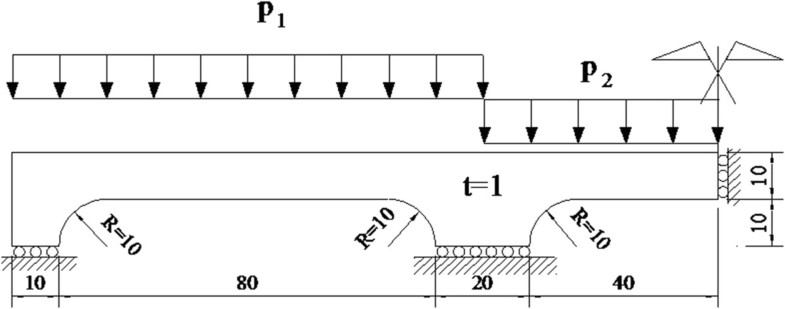Figure 3 Continuous beam.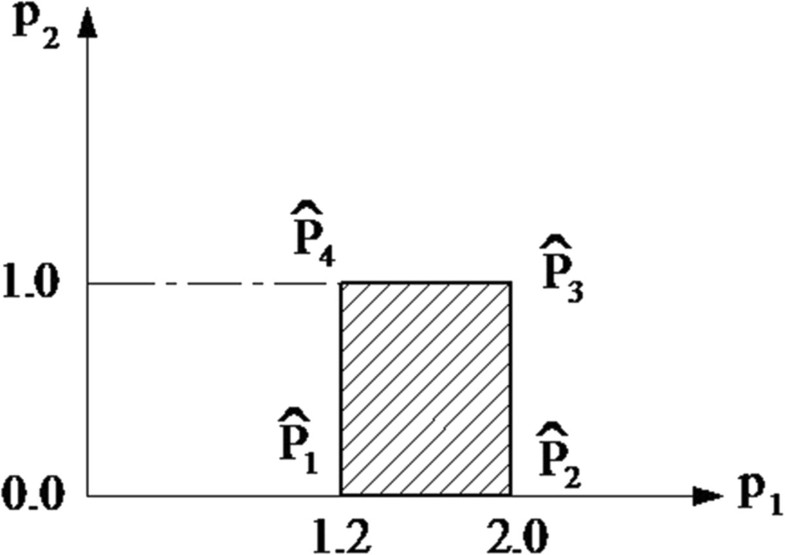Figure 4 Load domain for example 4.1.
The material mechanical properties are Young's modulus, E = 1.8 ⋅ 105 N/mm2; yield stress, σ y  = 100 N/mm2; ultimate strength, σ u  = 1.35σ y and Poisson's ratio, ν = 0.3. By the symmetry of the problem, only half of the structure is discretized into 589 elements, 8-node quadrangle, Figure 5. The structure is considered as a plane stress problem. Numerical limit and shakedown analysis for this structure made of perfectly plastic material were presented in Garcea et al.  and Tran et al. .Figure 5 FEM mesh.
Table 1 shows the results of limit and shakedown analysis. Present results are close to others in literature.
Table 1

Comparison of plastic limit collapse and shakedown results

Limit

Shakedown

Author

[p1, p2] = [2.0, 0.0]

[p1, p2] = [0.0, 1.0]

[p1, p2] = [1.2, 1.0]

[p1, p2] = [2.0, 1.0]

$\begin{array}{l}{\mathbit{p}}_{\mathbf{1}}\mathbf{\in }\left[\mathbf{1.2}\mathbf{,}\mathbf{2}\right]\\ {\mathbit{p}}_{\mathbf{2}}\mathbf{\in }\left[\mathbf{0}\mathbf{,}\mathbf{1}\right]\end{array}$

Garcea et al. 

3.280

8.718

5.467

3.280

3.244

Tran et al. 

3.402

9.192

5.720

3.388

3.377

Present (perfectly plastic)

3.300

8.744

5.500

3.300

3.264

Present (kin. hardening)

4.455

11.804

7.425

4.455

4.406

Interaction diagram of shakedown load multiplier is plotted in Figure 6. In this structure, when p2 is not very large, the structure fails in ratcheting mode, and benefit of hardening is quite clear.Figure 6 Interaction diagram for shakedown bounds of continuous beam. The results are not normalized.

This closed-end pipe is investigated for perfectly plastic material in Vu  using primal-dual shakedown algorithm. The structure is subjected to bending Mb and torsion Mt moments, internal pressure p and axial tension T. Material properties are Young's modulus, E = 2.1 ⋅ 105 N/mm2; yield stress, σ y  = 160 N/mm2; ultimate strength, σ u  = 1.25σ y and Poisson's ratio, ν = 0.3. Using 20-node 3D elements to model whole structure with the dimensions: length L = 2, 700 mm, mean radius r = 300 mm and thickness h = 60 mm, see Figure 7.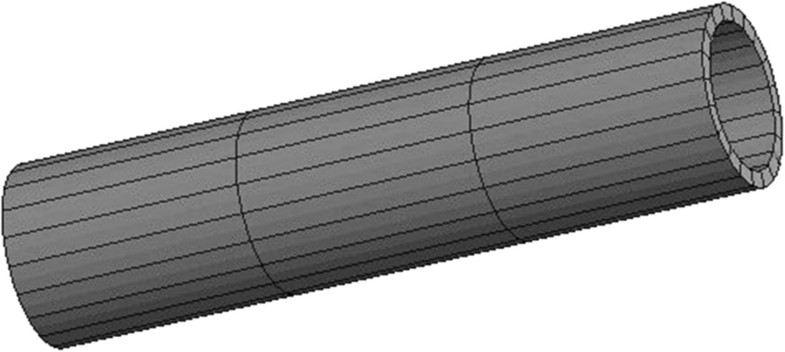Figure 7 FEM mesh of cylindrical pipe.

The analytical solutions of plastic collapse limit for cylindrical pipe under complex loading can be cited from Vu .

Pure bending capacity:
${\mathit{M}}_{\mathit{b}lim}=4{\mathit{\sigma }}_{\mathit{y}}\mathit{h}\left({\mathit{r}}^{2}+\frac{{\mathit{h}}^{2}}{12}\right)=3647.52\cdot {10}^{6}\phantom{\rule{0.25em}{0ex}}\mathrm{Nmm}.$
(30)
Pure torsion capacity:
${\mathit{M}}_{\mathit{t}lim}=\frac{2}{\sqrt{3}}\mathit{\pi }{\mathit{r}}^{2}\mathit{h}{\mathit{\sigma }}_{\mathit{y}}=3134.24\cdot {10}^{6}\phantom{\rule{0.25em}{0ex}}\mathrm{Nmm}.$
(31)
Pure tension capacity:
${\mathit{T}}_{\text{lim}}=2\mathit{\pi rh}{\mathit{\sigma }}_{\mathit{y}}=18095573.6\phantom{\rule{0.25em}{0ex}}\mathrm{N}.$
(32)
Pure internal pressure capacity:
${\mathit{p}}_{\text{lim}}={\mathit{\sigma }}_{\mathit{y}}\frac{\mathit{h}}{\mathit{r}}=32\phantom{\rule{0.25em}{0ex}}\mathrm{N}/{\mathrm{mm}}^{2}$
(33)
and the normalized load multiplier when bending, internal pressure and tension are combined is as follows:
$\mathit{m}=\frac{\sqrt{4-3{\mathit{n}}_{\mathit{\phi }}^{2}}}{2}cos\left[\frac{{\mathit{n}}_{\mathit{\phi }}-2{\mathit{n}}_{\mathit{x}}}{\sqrt{4-3{\mathit{n}}_{\mathit{\phi }}^{2}}}\frac{\mathit{\pi }}{2}\right],$
(34)
where
$\left\{\begin{array}{l}\mathit{m}=\mathit{M}/{\mathit{M}}_{\mathit{b}lim}\\ {\mathit{n}}_{\mathit{\phi }}=\mathit{p}/{\mathit{p}}_{\text{lim}}\\ {\mathit{n}}_{\mathit{x}}=\mathit{T}/{\mathit{T}}_{\text{lim}}\end{array}\right\.$
(35)
If the axial tension force comes from only internal pressure on closed ends, then n x  = n φ /2, and formula (35) can be rewritten as
$\mathit{m}=\frac{\sqrt{4-3{\mathit{n}}_{\mathit{\phi }}^{2}}}{2}$
(36)
FE analysis is fulfilled for structure subjected to combined internal pressure p and bending Mb. Results are presented in Table 2, normalized by pure bending capacity in formula (28) and pure internal pressure in formula (33). Limit analysis is implemented for σ u /σ y  = 1.0 to be compared to formula (36), and interaction diagram is plotted in Figure 8. Shakedown analysis with and without hardening effect is implemented for the load domain: p ∈ [0, 1]; M b  ∈ [−1, 1]. Interaction diagram is plotted in Figure 9.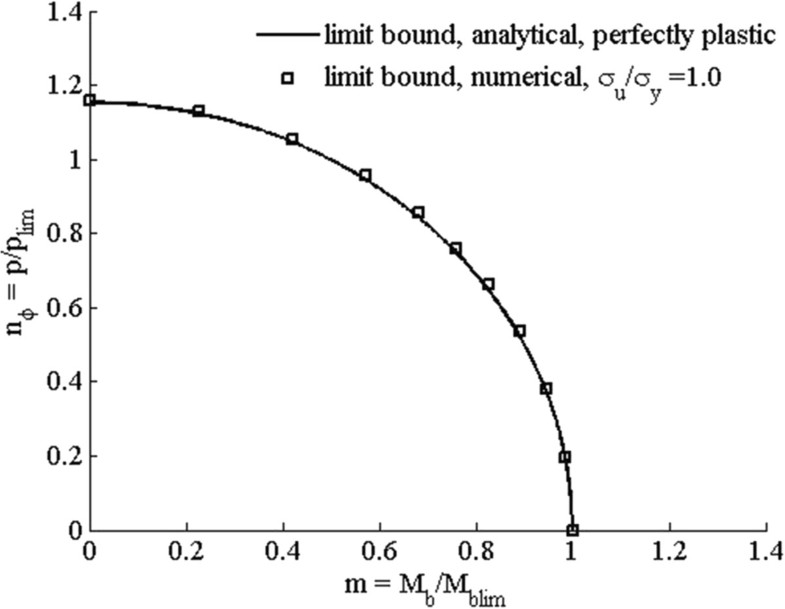Figure 8 Interaction diagram for limit bounds. Comparison between analytical and numerical solutions.Figure 9 Interaction diagram for elastic and shakedown bounds, normalized by pure plastic collapse limits, M b lim and p lim .
Table 2

Limit and shakedown load multipliers of cylindrical pipe subjected to internal pressure and bending

Elastic factor

Limit factor (perfectly plastic)

Shakedown factor (perfectly plastic)

Shakedown factor (bounded hardening)

Shakedown factor (unbounded hardening)

0.0p_1.0 M

0.7338

1.0012

0.7338

0.7338

0.7338

0.2p_1.0 M

0.7228

0.9870

0.7297

0.7310

0.7304

0.4p_1.0 M

0.7011

0.9478

0.7228

0.7236

0.7231

0.6p_1.0 M

0.6570

0.8914

0.7131

0.7132

0.7134

0.8p_1.0 M

0.6023

0.8267

0.7011

0.7013

0.7014

1.0p_1.0 M

0.5509

0.7608

0.6667

0.6855

0.6853

1.0p_0.8 M

0.6168

0.8540

0.7696

0.8128

0.8127

1.0p_0.6 M

0.6921

0.9556

0.8906

0.9894

0.9894

1.0p_0.4 M

0.7727

1.0546

1.0179

1.2318

1.2346

1.0p_0.2 M

0.8486

1.1306

1.1204

1.3874

1.5506

1.0p_0.0 M

0.9019

1.1589

1.1586

1.4482

1.8091

Figure 8 shows that the present results of limit analysis for σ u /σ y  = 1 are close to analytical solutions. Figure 9 shows that the hardening effect is clear if the applied moment is less than 0.5Mb lim. If σ u  ≥ 2σ y , bounded hardening model becomes unbounded, and shakedown limit of structure cannot exceed two times of elastic limit.

## Conclusions

The paper developed a new upper bound algorithm for shakedown analysis of elastic plastic-bounded linearly kinematic hardening structures. This is an efficient tool for practical computation, especially for complicated structures subject to mechanical loads.

The proposed algorithm gives results that are close to the results in literatures. If σ u  = σ y , it leads to perfectly plastic material; if σ u  ≥ 2σ y , it leads to unbounded kinematic hardening material; otherwise, σ y  < σ u  < 2σ y , we have bounded kinematic hardening material.

Let ${\mathit{\alpha }}_{\mathrm{el}},{\mathit{\alpha }}_{\mathrm{sd}}^{\mathrm{pp}},\mathrm{and}\phantom{\rule{0.25em}{0ex}}{\mathit{\alpha }}_{\mathrm{sd}}^{\mathrm{blkh}}$ denote respectively elastic limit, shakedown limit for elastic perfectly plastic and shakedown limit for bounded kinematic hardening material, respectively, then:
${\mathit{\alpha }}_{\mathrm{sd}}^{\mathrm{pp}}\phantom{\rule{0.2em}{0ex}}\le \phantom{\rule{0.2em}{0ex}}{\mathit{\alpha }}_{\mathrm{sd}}^{\mathrm{blkh}}\phantom{\rule{0.2em}{0ex}}\le \frac{{\mathit{\sigma }}_{\mathit{u}}}{{\mathit{\sigma }}_{\mathit{y}}}{\mathit{\alpha }}_{\mathrm{sd}}^{\mathrm{pp}}\le 2{\mathit{\alpha }}_{\mathrm{el}}.$

In the preceding expression, the left equality occurs if the subsequent yield surface translates inside the bounding surface, the middle equality occurs if the subsequent yield surface fixed on the bounding surface and the last equality occurs when yield surface translates unboundedly. If the structure shakes down in alternating plasticity mode, then there is no difference between perfectly plastic and kinematic hardening models.

## Supplementary material

40540_2013_4_MOESM1_ESM.tiff (352 kb)
Authors’ original file for figure 1
40540_2013_4_MOESM2_ESM.tiff (376 kb)
Authors’ original file for figure 2
40540_2013_4_MOESM3_ESM.tiff (227 kb)
Authors’ original file for figure 3
40540_2013_4_MOESM4_ESM.tiff (169 kb)
Authors’ original file for figure 4
40540_2013_4_MOESM5_ESM.tiff (754 kb)
Authors’ original file for figure 5
40540_2013_4_MOESM6_ESM.tiff (200 kb)
Authors’ original file for figure 6
40540_2013_4_MOESM7_ESM.tiff (569 kb)
Authors’ original file for figure 7
40540_2013_4_MOESM8_ESM.tiff (191 kb)
Authors’ original file for figure 8
40540_2013_4_MOESM9_ESM.tiff (237 kb)
Authors’ original file for figure 9

## References

1. 1.
Melan E: Zur Plastizität des räumlichen Kontinuums. Ing-Arch 1938, 8: 116–126.
2. 2.
Prager W: A new method of analyzing stress and strain in work hardening plastic solids. J Appl Mech ASME 1956, 23: 493–496.
3. 3.
Maier GA: Shakedown matrix theory allowing for work hardening and second-order geometric effects. In Foundations of plasticity. Edited by: Sawczuk A. North-Holland, Amsterdam: Springer; 1973:417–433.Google Scholar
4. 4.
Ponter ARS: A general shakedown theorem for elastic plastic bodies with work hardening. Proc. SMiRT-3, paper L5/2 1975.Google Scholar
5. 5.
Gokhfeld DA, Cherniavsky OF: Limit analysis of structures at thermal cycling. The Netherlands: Sijthoff & Noordhoff; 1980.Google Scholar
6. 6.
Stein E, Huang YJ: Shakedown for systems of kinematic hardening materials. In Inelastic behaviour of structures under variables loads. Edited by: Weichert D, Doroz S, Mróz Z. Springer, Netherlands: Kluwer Academic Publishers; 1995:33–50.
7. 7.
Weichert D, Groß-Weege J: The numerical assessment of elastic-plastic sheets under variable mechanical and thermal loads using a simplified two-surface yield condition. Int J Mech Sci 1988, 30: 757–767. 10.1016/0020-7403(88)90040-9
8. 8.
Bodovillé G, de Saxcé G: Plasticity with non-linear kinematic hardening–modelling and shakedown analysis by the bipotential approach. Eur J Mech A/Solids 2001, 20: 99–112. 10.1016/S0997-7538(00)01109-8
9. 9.
Pham DC: Shakedown static and kinematic theorems for elastic-plastic limited linear kinematic-hardening solids. Eur J Mech A/Solids 2005, 24: 35–45. 10.1016/j.euromechsol.2004.11.001
10. 10.
Pham DC: Shakedown theory for elastic plastic kinematic hardening bodies. Int J Plast 2007, 23: 1240–1259. 10.1016/j.ijplas.2006.11.003
11. 11.
Nguyen QS: On shakedown analysis in hardening. J Mech Phys Solids 2003, 51: 101–125. 10.1016/S0022-5096(02)00058-3
12. 12.
Staat M, Heitzer M: The restricted influence of kinematic hardening on shakedown loads. Vienna, Austria: Proceedings of WCCM V, 5th World Congress on Computational Mechanics; 2002. http://opus.bibliothek.fh-aachen.de/opus/volltexte/2005/79/Google Scholar
13. 13.
Staat M, Heitzer M (Eds): NIC Series In Numerical Methods for Limit and Shakedown analysis–Deterministic and Probabilistic Problems. Volume 15. Jülich: John von Neumann Institute for Computing; 2003. http://webarchiv.fz-juelich.de/nic-series/volume15/volume15.html
14. 14.
Stein E, Zhang G: Theoretical and numerical shakedown analysis for kinematic hardening materials. In Proc. 3rd Int. Conf. on Computational Plasticity (COMPLAS 3), CIMNE. Edited by: Owen DRJ, Oñate E, Hinton E. Barcelona, Spain: Pineridge Press; 1992:1–25.Google Scholar
15. 15.
Yan AM, Nguyen Dang H: Direct finite element kinematical approaches in limit and shakedown analysis of shells and elbows. In Inelastic Analysis of Structures under variable Loads, Theory and Engineering Applications. Springer, Netherlands: Kluwer Academic Publishers; 2000:233–254.
16. 16.
Yan AM, Nguyen Dang H: Kinematical shakedown analysis with temperature-dependent yield stress. Int J Num Mech Engng 2001, 50: 1415–1168.Google Scholar
17. 17.
Yan AM, Khoi VD, Nguyen Dang H: Kinematical formulation of limit and shakedown analysis. NIC Series. In Numerical Methods for Limit and Shakedown Analysis–Deterministic and Probabilistic Problems. Volume 15. Jülich: John von Neumann Institute for Computing; 2003. http://webarchiv.fz-juelich.de/nic-series/volume15/volume15.htmlGoogle Scholar
18. 18.
Nguyen Dang H, König J: A finite element formulation for shakedown problems using a yield criterion of the mean. Comp Appl Mech Eng 1976,1(Nr. 2):179–182.
19. 19.
Nguyen Dang H, Palgen L: Shakedown analysis by displacement method and equilibrium finite element. Transac CSME 1980–81,6(Nr. 1):32–39.Google Scholar
20. 20.
Vu DK, Yan AM, Nguyen Dang H: A dual form for discretized kinematic formulation in shakedown analysis. Int J Solids Struct 2003,41(1):267–277.Google Scholar
21. 21.
Vu DK, Yan AM, Nguyen Dang H: A primal-dual algorithm for shakedown analysis of structures. Comp Methods Appl Mech Eng (Elsevier) 2004,193(42–44):4663–4674.
22. 22.
Yan AM, Vu DK, Nguyen Dang H: Dual in kinematical approaches of limit and shakedown analysis of structures. In Complimentarily, duality and symmetry in nonlinear mechanics. Volume 6. Edited by: David Y. Springer, Netherlands: Gao, Kluwer Academic Publishers; 2004:127–148. 10.1007/978-90-481-9577-0_7
23. 23.
Vu DK: Dual Limit and Shakedown analysis of structures. PhD Thesis. Belgium: Université de Liège; 2001.Google Scholar
24. 24.
Phạm PT, Staat M, et al.: An upper bound algorithm for limit and shakedown analysis of bounded linearly kinematic hardening bodies. In Direct Methods. Edited by: De Saxcé G. Netherlands: Springer; 2013.Google Scholar
25. 25.
Garcea G, Armentano G, Petrolo S, Casciaro R: Finite element shakedown of two-dimensional structures. Int J Numer Mech Engng 2005, 63: 1174–1202. 10.1002/nme.1316
26. 26.
Tran TN, Liu GR, Nguyen XH, Nguyen TT: An edge-based smoothed finite element method for primal-dual shakedown analysis of structures. Int J Numer Engng 2010, 82: 917–938.Google Scholar